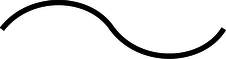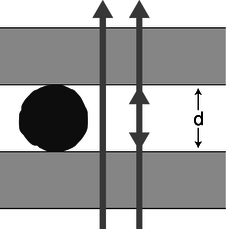# 7.4: Problems

$$\newcommand{\vecs}{\overset { \rightharpoonup} {\mathbf{#1}} }$$ $$\newcommand{\vecd}{\overset{-\!-\!\rightharpoonup}{\vphantom{a}\smash {#1}}}$$$$\newcommand{\id}{\mathrm{id}}$$ $$\newcommand{\Span}{\mathrm{span}}$$ $$\newcommand{\kernel}{\mathrm{null}\,}$$ $$\newcommand{\range}{\mathrm{range}\,}$$ $$\newcommand{\RealPart}{\mathrm{Re}}$$ $$\newcommand{\ImaginaryPart}{\mathrm{Im}}$$ $$\newcommand{\Argument}{\mathrm{Arg}}$$ $$\newcommand{\norm}{\| #1 \|}$$ $$\newcommand{\inner}{\langle #1, #2 \rangle}$$ $$\newcommand{\Span}{\mathrm{span}}$$ $$\newcommand{\id}{\mathrm{id}}$$ $$\newcommand{\Span}{\mathrm{span}}$$ $$\newcommand{\kernel}{\mathrm{null}\,}$$ $$\newcommand{\range}{\mathrm{range}\,}$$ $$\newcommand{\RealPart}{\mathrm{Re}}$$ $$\newcommand{\ImaginaryPart}{\mathrm{Im}}$$ $$\newcommand{\Argument}{\mathrm{Arg}}$$ $$\newcommand{\norm}{\| #1 \|}$$ $$\newcommand{\inner}{\langle #1, #2 \rangle}$$ $$\newcommand{\Span}{\mathrm{span}}$$$$\newcommand{\AA}{\unicode[.8,0]{x212B}}$$

1. The musical note middle C has a frequency of 262 Hz. What are its period and wavelength?

2. The following is a graph of the height of a water wave as a function of position, at a certain moment in time.Trace this graph onto another piece of paper, and then sketch below it the corresponding graphs that would be obtained if

(a) the amplitude and frequency were doubled while the velocity remained the same;

(b) the frequency and velocity were both doubled while the amplitude remained unchanged;

(c) the wavelength and amplitude were reduced by a factor of three while the velocity was doubled.a / Problem 3

3. (a) The graph shows the height of a water wave pulse as a function of position. Draw a graph of height as a function of time for a specific point on the water. Assume the pulse is traveling to the right.
(b) Repeat part a, but assume the pulse is traveling to the left.
(c) Now assume the original graph was of height as a function of time, and draw a graph of height as a function of position, assuming the pulse is traveling to the right.
(d) Repeat part c, but assume the pulse is traveling to the left.

4. At a particular moment in time, a wave on a string has a shape described by $$y=3.5\cos (0.73\pi x+0.45\pi t+0.37\pi)$$. The stuff inside the cosine is in radians. Assume that the units of the numerical constants are such that $$x$$, $$y$$, and $$t$$ are in SI units.\hwhint{hwhint:sinwavekinem}
(a) Is the wave moving in the positive $$x$$ or the negative $$x$$ direction?
(b) Find the wave's period, frequency, wavelength.
(c) Find the wave's velocity.
(d) Find the maximum velocity of any point on the string, and compare with the magnitude and direction of the wave's velocity. (answer check available at lightandmatter.com)b / Problem 5.

5. The figure shows one wavelength of a steady sinusoidal wave traveling to the right along a string. Define a coordinate system in which the positive $$x$$ axis points to the right and the positive $$y$$ axis up, such that the flattened string would have $$y=0$$. Copy the figure, and label with $$y=0$$ all the appropriate parts of the string. Similarly, label with $$v=0$$ all parts of the string whose velocities are zero, and with $$a=0$$ all parts whose accelerations are zero. There is more than one point whose velocity is of the greatest magnitude. Pick one of these, and indicate the direction of its velocity vector. Do the same for a point having the maximum magnitude of acceleration. Explain all your answers.

[Problem by Arnold Arons.]

6. Find an equation for the relationship between the Doppler-shifted frequency of a wave and the frequency of the original wave, for the case of a stationary observer and a source moving directly toward or away from the observer.

7. Suggest a quantitative experiment to look for any deviation from the principle of superposition for surface waves in water. Try to make your experiment simple and practical.c / Problem 8.

8. The simplest trick with a lasso is to spin a flat loop in a horizontal plane. The whirling loop of a lasso is kept under tension mainly due to its own rotation. Although the spoke's force on the loop has an inward component, we'll ignore it. The purpose of this problem, which is based on one by A.P. French, is to prove a cute fact about wave disturbances moving around the loop. As far as I know, this fact has no practical implications for trick roping! Let the loop have radius $$r$$ and mass per unit length $$\mu$$, and let its angular velocity be $$\omega$$.
(a) Find the tension, $$T$$, in the loop in terms of $$r$$, $$\mu$$, and $$\omega$$. Assume the loop is a perfect circle, with no wave disturbances on it yet. \hwhint{hwhint:lasso} \hwans{hwans:lasso}
(b) Find the velocity of a wave pulse traveling around the loop. Discuss what happens when the pulse moves is in the same direction as the rotation, and when it travels contrary to the rotation.

9. A string hangs vertically, free at the bottom and attached at the top.
(a) Find the velocity of waves on the string as a function of the distance from the bottom
(b) Find the acceleration of waves on the string.\hwans{hwans:wave-on-hanging-string}
(c) Interpret your answers to parts a and b for the case where a pulse comes down and reaches the end of the string. What happens next? Check your answer against experiment and conservation of energy.

10. Singing that is off-pitch by more than about 1% sounds bad. How fast would a singer have to be moving relative to a the rest of a band to make this much of a change in pitch due to the Doppler effect?

11. Light travels faster in warmer air. Use this fact to explain the formation of a mirage appearing like the shiny surface of a pool of water when there is a layer of hot air above a road.

12. (a) Compute the amplitude of light that is reflected back into air at an air-water interface, relative to the amplitude of the incident wave. Assume that the light arrives in the direction directly perpendicular to the surface.The speeds of light in air and water are $$3.0\times10^8$$ and $$2.2\times10^8$$ m/s, respectively.
(b) Find the energy of the reflected wave as a fraction of the incident energy. \hwhint{hwhint:airwaterrefl}(answer check available at lightandmatter.com)

13. A concert flute produces its lowest note, at about 262 Hz, when half of a wavelength fits inside its tube. Compute the length of the flute. \hwans{hwans:flute}

14. (a) A good tenor saxophone player can play all of the following notes without changing her fingering, simply by altering the tightness of her lips: E$$\flat$$ (150 Hz), E$$\flat$$ (300 Hz), B$$\flat$$ (450 Hz), and E$$\flat$$ (600 Hz). How is this possible? (I'm not asking you to analyze the coupling between the lips, the reed, the mouthpiece, and the air column, which is very complicated.)
(b) Some saxophone players are known for their ability to use this technique to play “freak notes,” i.e., notes above the normal range of the instrument. Why isn't it possible to play notes below the normal range using this technique?

 C 261.6 Hz D 293.7 E 329.6 F 349.2 G 392.0 A 440.0 flat 466.2

Problem 15.

15. The table gives the frequencies of the notes that make up the key of F major, starting from middle C and going up through all seven notes.
(a) Calculate the first four or five harmonics of C and G, and determine whether these two notes will be consonant or dissonant. (Recall that harmonics that differ by about 1-10% cause dissonance.)
(b) Do the same for C and B$$\flat$$.d / Problem 16.

16. A Fabry-Perot interferometer, shown in the figure being used to measure the diameter of a thin filament, consists of two glass plates with an air gap between them. As the top plate is moved up or down with a screw, the light passing through the plates goes through a cycle of constructive and destructive interference, which is mainly due to interference between rays that pass straight through and those that are reflected twice back into the air gap. (Although the dimensions in this drawing are distorted for legibility, the glass plates would really be much thicker than the length of the wave-trains of light, so no interference effects would be observed due to reflections within the glass.)
(a) If the top plate is cranked down so that the thickness, $$d$$, of the air gap is much less than the wavelength $$\lambda$$ of the light, i.e., in the limit $$d \rightarrow 0$$, what is the phase relationship between the two rays? (Recall that the phase can be inverted by a reflection.) Is the interference constructive, or destructive?
(b) If $$d$$ is now slowly increased, what is the first value of $$d$$ for which the interference is the same as at $$d\rightarrow 0$$? Express your answer in terms of $$\lambda$$.
(c) Suppose the apparatus is first set up as shown in the figure. The filament is then removed, and $$n$$ cycles of brightening and dimming are counted while the top plate is brought down to $$d=0$$. What is the thickness of the filament, in terms of $$n$$ and $$\lambda$$?
Based on a problem by D.J. Raymond.

17. (a) A wave pulse moves into a new medium, where its velocity is greater by a factor $$\alpha$$. Find an expression for the fraction, $$f$$, of the wave energy that is transmitted, in terms of $$\alpha$$. Note that, as discussed in the text, you cannot simply find $$f$$ by squaring the amplitude of the transmitted wave. \hwans{hwans:maxtransmission}
(b) Suppose we wish to transmit a pulse from one medium to another, maximizing the fraction of the wave energy transmitted. To do so, we sandwich another layer in between them, so that the wave moves from the initial medium, where its velocity is $$v_1$$, through the intermediate layer, where it is $$v_2$$, and on into the final layer, where it becomes $$v_3$$. What is the optimal value of $$v_2$$? (Assume that the middle layer is thicker than the length of the pulse, so there are no interference effects. Also, although there will be later echoes that are transmitted after multiple reflections back and forth across the middle layer, you are only to optimize the strength of the transmitted pulse that is first to emerge. In other words, it's simply a matter of applying your answer from part a twice to find the amount that finally gets through.) \hwans{hwans:maxtransmission}

(c) 1998-2013 Benjamin Crowell, licensed under the Creative Commons Attribution-ShareAlike license. Photo credits are given at the end of the Adobe Acrobat version.